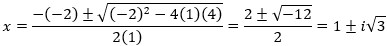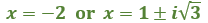Factor and Solve Polynomial Equations

Factor and Solve Polynomial Equations

Goal: Solving polynomial equations by factoring.

Polynomial Identities

In order to solve polynomials we need to be aware of basic identities which allow us to simplify the equation.

The following are the main identities for binomial factors

1.) x2 y2 = (x  y)(x + y)
Example:  9  p2  =  (3  p)(3 + p)

2.) x3 + y3 = (x + y)(x2
xy + y2)
Example:  64 + 8x3  =  (4 + 2x)(16  8x  + 4x2)

3.)
x3  y3 = (x  y)(x2 + xy + y2)
Example:  64 − 8x3  =  (4  2x)(16 + 8x + 4x2)

4.)
x4  y4 = (x y)(x + y)(x2 + y2)
Example:  81y4  625x8  =  (3y  5x2)(3y + 5x2)(9y2 + 25x4)

Solve by Factoring

Example

Solve for x in the equation by factoring and find all the complex roots:  2x3 + 16 = 0

Solution

Since 2 is a common factor to each term, factor out 2:

2(x3 + 8) = 0

Then factor the remaining cubic expression:

2(x + 2)(x2  2x + 4) = 0

Use the zero-product property:

2 = 0   OR   x + 2 = 0   OR   x2 − 2x + 4 = 0

Solve each equation:

2 ≠ 0, so this one gets discarded

x  =  0 − 2  =  −2and we find the solutions